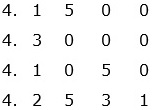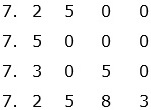# Ordering Decimals

#### Complete Python Prime Pack

9 Courses     2 eBooks

#### Artificial Intelligence & Machine Learning Prime Pack

6 Courses     1 eBooks

#### Java Prime Pack

9 Courses     2 eBooks

### Rules for ordering decimals

• When comparing decimal numbers and arranging them in order we line up the numbers vertically with the decimal points in a vertical line.

• If a number doesn’t have a decimal point, we place the decimal at the end.

• We then fill in blanks with zeroes to make the columns easier to line up.

• We start comparing the digits of numbers from the left and come to the leftmost unlike digits and compare them to decide which of the two decimal numbers is lesser or greater.

• Comparing two decimal numbers at a time, we group the given decimal numbers either increasing or decreasing as required.

Order the numbers below from least to the greatest

4.15, 4.3, 4.105, 4.2531

### Solution

Step 1:

Rewriting the numbers with same number of decimal places, we get 4.1500; 4.3000; 4.1050 and 4.2531
Lining them up vertically with their decimal points one above another.Step 2:

We see that all numbers have the same digit 4 in ones place.

In tenths digit 1 is minimum and 3 is maximum. So 4.3000 is the biggest number.

The next smaller number is 4.2531.

Step 3:

There are two numbers with 1 in tenths place.

We compare these two now. In hundredth place there is digit 5 in 4.1500 and 0 in 4.1050. So 4.1050 < 4.1500

Step 4:

So, the numbers ordered from least to greatest are

4.1050 < 4.1500 < 4.2531 < 4.3000

Order the numbers below from least to the greatest

7.25, 7.5, 7.305, 7.2583

### Solution

Step 1:

Rewriting the numbers with same number of decimal places we get 7.2500; 7.5000; 7.3050 and 7.2583. We line them up as shown belowStep 2:

We see that all numbers have the same digit 7 in ones place.

In tenths digit 2 is minimum and 5 is maximum. So 7.5 is the biggest number.

The next smaller number is 7.3050.

Step 3:

There are two numbers with 2 in tenths place.

We compare these two now. These have same digit 5 in hundredth place.

In thousandth place there is digit 8 in 7.2583 and 0 in 7.2500. So 7.25 < 7.2583

Step 4:

So the numbers ordered from least to greatest are

7.25 < 7.2583 < 7.3050 < 7.5